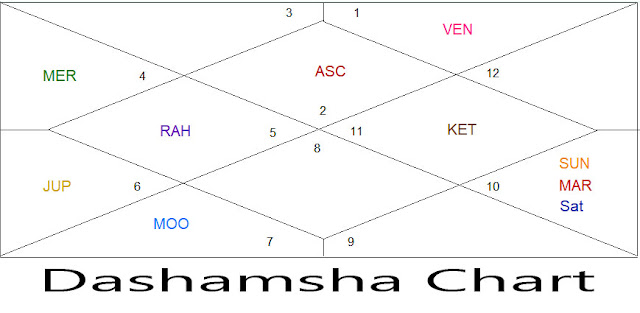How to Calculate Dashamsha (D-10) ?

Dashamsha chart is a very important sub-division of natal chart in vedic astrology. Dashamsha chart is used to look karma of native. Almost every astrologer likes to look Dashamsha  chart with natal Chart to study Career, success, Profession, Professional achievement, Promotion, Demotion, Self-Earned Money etc.
Tenth division of a rasi is known as a Dashamsha .
1 Dashamsha = 300/10 = 30

Steps to calculate Dashamsha.

We have to take degree of a planet and divide it by 30, If the answer is in decimal, we have to round up the answer.
1)     If the planet is in Odd sign in natal Chart, we will start counting from the sign where planet is situated up to the number which we have found from the division of planet degree by 30, In Dashamsha chart we will put the planet where the counting ends.

2)     If the planet is in Even sign in natal Chart, we will start counting from the 9th sign where planet is situated up to the number which we have found from the division of planet degree by 30, In Dashamsha chart we will put the planet where the counting ends.In natal Chart Ascendant ( Lagna ) is in Mesh ( Aries ) at 3023’

So according to above mentioned rule we have to divide ascendant degree by 30
= 3023’/30’ = 1+ then we will round up the number that is = 2
Because Mesh ( Aries ) is an odd sign we will start counting from Mesh (Aries) to 2nd  houses, so we will find vrisha ( Taurus ) that means in Dashamsha Chart Ascendant will fall in Vrisha Rashi ( Taurus ).

Let’s take Rahu which is in Kanya ( Virgo ) at 9053’
So, we divide the Rahu degree by 30
= 9053’ / 30’ = 3 + then we will round up the number that is = 4
Virgo is an Even sign so we will count 4th  from the 9th  of Virgo which is Simha ( Leo ). Rahu will fall in Meen ( Pisces ) in Simha ( Leo ).

Let’s take Venus which is at Libra ( Tula ) at 20027’
So, we divide 20027’ / 30 = 6+ then we will round up the number that is = 7
Tula is an odd sign so according to above mentioned formula we will start counting from Tula and 7th from Tula is Aries ( Mesha ), We will place Venus in Aries ( Mesha ).

Let’s take Sun which is at Dhanu ( Sagittarius ) at 5047’
So, we divide 5047’ / 30 = 1+ then we will round up the number that is 2
Dhanu is an odd sign so we will put sun 2nd from Dhanu which is Makara (Capricon).

Let’s take Mercury which is at Dhanu ( Sagittarius )  at 21050’
So, we divide 21050’ / 30 = 7+ then we will round up the number that is = 8
Dhanu is an odd sign so we will put Mercury 8th from Dhanu which is Karka (Cancer).

Let’s take Moon which is at Makara (Capricon) at 3021’
So, we divide 3021’ / 30 = 1+ then round up the number which is 2
Makara is an even sign so we will put Moon 2nd from the 9th of Makara which is Tula ( Venus ).

Table of Dashamsha ( Tenth Division )

 Deg Ari Tau Gem Can Leo Vir Lib Sco Sag Cap Aqu Pis 00-30 Ari Cap Gem Pis Leo Tau Lib Can Sag Vir Aqu Sco 30-60 Tau Aqu Can Ari Vir Gem Sco Leo Cap Lib Pis Sag 60-90 Gem Pis Leo Tau Lib Can Sag Vir Aqu Sco Ari Cap 90-120 Can Ari Vir Gem Sco Leo Cap Lib Pis Sag Tau Aqu 120-150 Leo Tau Lib Can Sag Vir Aqu Sco Ari Cap Gem Pis 150-180 Vir Gem Sco Leo Cap Lib Pis Sag Tau Aqu Can Ari 180-210 Lib Can Sag Vir Aqu Sco Ari Cap Gem Pis Leo Tau 210-240 Sco Leo Cap Lib Pis Sag Tau Aqu Can Ari Vir Gem 240-270 Sag Vir Aqu Sco Ari Cap Gem Pis Leo Tau Lib Can 270-300 Cap Lib Pis Sag Tau Aqu Can Ari Vir Gem Sco Leo

This is a simplified chart for Dashamsha Calculation.

If Planet is in between 00 to 30 of Mesha Rasi in Natal Chart then in  Dashamsha chart planet will placed in Mesha Rasi.

If Planet is in between 30 to 60 of Mesha Rasi in Natal Chart then in Dashamsha Chart Planet will placed in Vrisha Rasi.

If Planet is in between 00 to 30 of Vrisha Rasi in Natal Chart then in  Dashamsha chart planet will placed in Capricon Makara Rasi.

If Planet is in between 00 to 30 of Mithuna Rasi in Natal Chart then in  Dashamsha chart planet will placed in Mithuna Rasi.